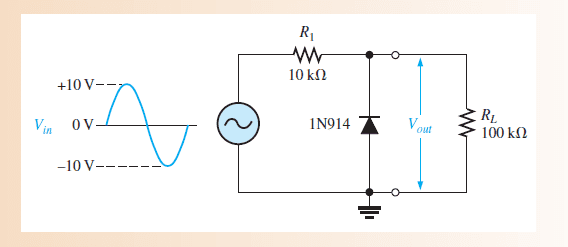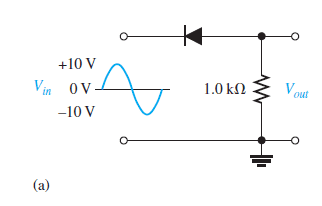# A problem with diode limitters

hello guys

while studing the diode limitters

i found two examples i solved the first one but i couldn't with the second
1 -2-in the first i know that the diode while be forward biased in the negative half of the signal
so the negative half will be limitted to -0.7 v
and the V peak output will be = [(RL/(RL+R1)]*Vinput = 9.09v

but i don't know what will be the difference between the first and the second problem !!

berkeman
Mentor
In the second one, for + input signal, will the diode be forward or reverse biased? What does that mean about current through the resistor?

For - input signal, ask the same questions...

In the second one, for + input signal, will the diode be forward or reverse biased? What does that mean about current through the resistor?

For - input signal, ask the same questions...

okay in the + input the diode will be reverse biased so the current will not pass , but in the case of - input the diode will be forward biased and the current will pass

am i right ??

berkeman
Mentor
okay in the + input the diode will be reverse biased so the current will not pass , but in the case of - input the diode will be forward biased and the current will pass

am i right ??

Correct. And V=IR, so you know when you will have an output voltage. Good job!Correct. And V=IR, so you know when you will have an output voltage. Good job!okay i know that , but i still don't know how will i calculate Vout

i think Vout will be = -10+0.7= -9.3v .. is that right ??

berkeman
Mentor
okay i know that , but i still don't know how will i calculate Vout

i think Vout will be = -10+0.7= -9.3v .. is that right ??

That would be the negative peak voltage. The output waveform will follow the input waveform (minus the diode drop) when the diode is conducting.

That would be the negative peak voltage. The output waveform will follow the input waveform (minus the diode drop) when the diode is conducting.

you mean that the output waveform will equal zero in the positive half and in the negative half it will equal -10v-0.7v ?? or what ??

berkeman
Mentor
you mean that the output waveform will equal zero in the positive half and in the negative half it will equal -10v-0.7v ?? or what ??

In the negative half cycle, the output voltage equals the input voltage, minus the diode drop. Sketch that on top of the input waveform plot...